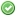rfc:const_scalar_exprs

# PHP RFC: Constant Scalar Expressions

## Introduction

This RFC brings static scalar expressions to the parser. This allows places that only take static values (const declarations, property declarations, function arguments, etc) to also be able to take static expressions.

This can allow for writing far easier to understand code, by allowing for far more expressive code.

The main difference to Anthony's RFC is (apart from a few operators more) that constants can be involved in these scalar operations:

const_scalar_exprs_diff.php
```<?php

const a = 1;

const b = a?2:100; // here the value of the constant "b" is dependent on the constant "a"

?>```

## Proposal

Adding parser support for scalar expressions: operations on constants or constant values.

### Supported Operations

The following operations are currently supported by this proposal:

• - - Subtraction
• * - Multiplication
• / - Division
• % - Modulus
• ! - Boolean Negation
• ~ - Bitwise Negation
• | - Bitwise OR
• & - Bitwise AND
• ^ - Bitwise XOR
• << - Bitwise Shift Left
• >> - Bitwise Shift Right
• . - Concatenation
• ?: - Ternary Operator
• <= - Smaller or Equal
• => - Greater or Equal
• == - Equal
• != - Not Equal
• < - Smaller
• > - Greater
• === - Identical
• !== - Not Identical
• && / and - Boolean AND
• || / or - Boolean OR
• xor - Boolean XOR

Also supported is grouping static operations: (1 + 2) * 3.

### Supported Operands

• 123 - Integers
• 123.456 - Floats
• “foo” - Strings
• __LINE__ - Line magic constant
• __FILE__ - File magic constant
• __DIR__ - Directory magic constant
• __TRAIT__ - Trait magic constant
• __METHOD__ - Method magic constant
• __FUNCTION__ - Function magic constant
• __NAMESPACE__ - Namespace magic constant
• <<<HEREDOC - HEREDOC string syntax (without variables)
• <<<'NOWDOC' - NOWDOC string syntax
• SOME_RANDOM_CONSTANT - Constants
• class_name::SOME_CONST - Class constants

### Constant Declarations

constant_declarations.php
```<?php
const FOO = 1 + 1;
const BAR = 1 << 1;
const GREETING = "HELLO";
const BAZ = GREETING." WORLD!"
?>```

### Class Constant Declarations

class_constant_declarations.php
```<?php
class Foo {
const FOO = 1 + 1;
const BAR = 1 << 1;
const GREETING = "HELLO";
const BAZ = self::GREETING." WORLD!"
}
?>```

### Class Property Declarations

class_property_declarations.php
```<?php
class Foo {
const BAZ = 10;
}

class Bar {
public \$foo = 1 + 1;
public \$bar = [
1 + 1,
1 << 2,
Foo::BAZ => "foo "."bar"
];
public \$baseDir = __DIR__ . "/base";
}
?>```

### Function Argument Declarations

function_argument_declarations.php
```<?php
const BAR = 1;

function foo(\$a = 1 + 1, \$b = 2 << 3, \$c = BAR?10:100) {
}
?>```

### Static Variable Declarations

static_variable_declarations.php
```<?php
const BAR = 0x10;

function foo() {
static \$a = 1 + 1;
static \$b = [1 << 2];
static \$c = 0x01 | BAR;
}
?>```

None

PHP 5.NEXT

## Patches and Tests

An implementation based off of current master is available: Implementation On GitHub (Diff On GitHub)

The patch is ready to be merged. (Opcache support is included, thanks to Dmitry)

## Note on implementation

The implementation of the scalar expressions is based on an AST. That AST implementation eventually could be used later as a general-purpose AST for compiler with a few tweaks.

## Vote

Should the patch for this RFC be merged into PHP 5.6?
Real name Yes No
ajf (ajf)bukka (bukka)bwoebi (bwoebi)datibbaw (datibbaw)dm (dm)indeyets (indeyets)juliens (juliens)krakjoe (krakjoe)laruence (laruence)levim (levim)mike (mike)pierrick (pierrick)reeze (reeze)seld (seld)treffynnon (treffynnon)tyrael (tyrael)willfitch (willfitch)yohgaki (yohgaki)Final result: 16 2
This poll has been closed.

The vote started the 20th November 2013 and ended the 27th November 2013.